Positional Astronomy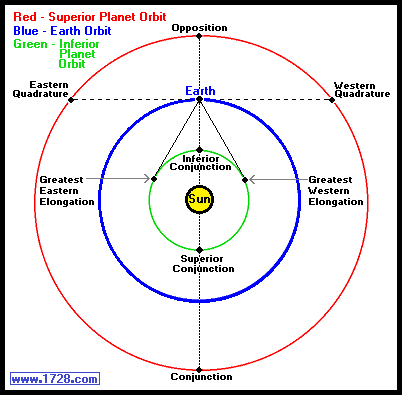As was mentioned in the Synodic Period Calculator, this is the time period required for a planet to return to the same point in its orbit relative to the Earth and Sun. For example, it can be the time between two conjunctions, the time between two oppositions, etc. Intuitively, you would think that this takes place in greater than one but less than two orbits of the inner planet and generally speaking you would be right. For example, the planet Neptune orbits the Sun in 60,190.03 days (164.79 years) and the Earth requires 365.25 days (1 year). Inputting this information into the synodic period calculator, we find that Neptune's synodic period is 367.48 days or 1.0061 years.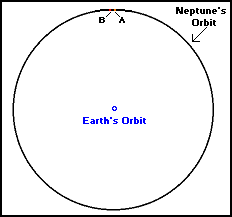Looking at the image above, in the time it takes the Earth to make slightly more than one orbit (about 362º), the planet Neptune moves from point A to point B (about 2º). Moving closer to the Sun, we see the planet Jupiter has an orbital period of 4,332.59 days (11.8618 years) and using the calculator, we see that Jupiter's synodic period is 398.88 days (1.0921 years).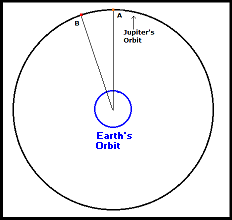Looking at the above graphic, we see that as Jupiter moves about 33º from point A to point B (the time of one synodic period), the Earth completes slightly more than one orbit (about 393º). So far, the planets we have examined have orbits in which the Earth only has to travel a little more than one revolution from one synodic period to the next. Looking at the graphic below, the orbit of Mars reveals an interesting phenomenon. At the Year 1 graphic, the Earth has completed one orbit and Mars has completed about 190° of its orbit. Looking at the Year 2 graphic, The Earth has completed two orbits and Mars finished a little over one orbit. In 2.135 years the planets are once again at opposition.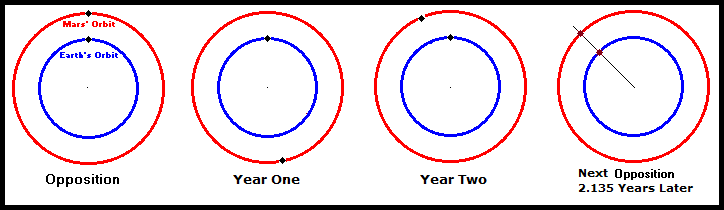It might seem somewhat contradictory that a faster planet can make two orbits without overtaking the slower planet but that is what just happened. When dealing with planetary orbits, the inner planet and outer planet can each make more than two orbits between synodic periods. For example look below at this graphic: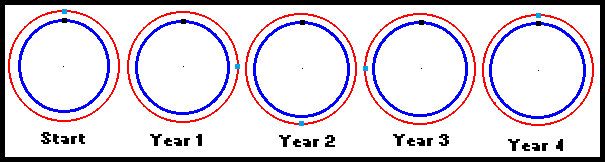The inner planet, Earth, makes four orbits per year and a fictional outer planet makes ¾ orbits per year or one orbit in 1⅓ years. Entering 1 and 1⅓ into the calculator yields a synodic period of four years. In that time the slower, outer planet makes three orbits while the faster, inner planet completes four. The general rule when dealing with all synodic period calculations, is that the outer planet will always make one less orbit than the inner planet.